# Sum and ratio

The sum and ratio of two numbers is equal to 10. Which numbers are they?

a =  9.0909
b =  0.9091

### Step-by-step explanation:

a+b = 10
a = 10b

a+b = 10
a = 10•b

a+b = 10
a-10b = 0

a = 100/11 ≈ 9.090909
b = 10/11 ≈ 0.909091

Our linear equations calculator calculates it.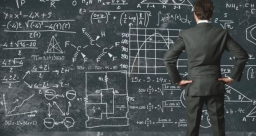Did you find an error or inaccuracy? Feel free to write us. Thank you!Tips to related online calculators
Do you have a system of equations and looking for calculator system of linear equations?

## Related math problems and questions:

• Three ints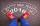The sum of three consecutive integers is 2016. What numbers are they?
• Ratio of two unknown numbersTwo numbers are given. Their sum is 30. We calculate one-sixth of a larger number and add to both numbers. So we get new numbers whose ratio is 5:7. Which two numbers were given?
• Two numbers 7The sum of two consecutive even numbers is 30. Find the numbers.
• Find the sumFind the sum of all natural numbers from 1 and 100, which are divisible by 2 or 5
• Big numbersHow many natural numbers less than 10 to the sixth can be written in numbers: a) 9.8.7 b) 9.8.0
• Two numbersThe sum of two numbers is 1. Identify this two numbers if you know that the half of first is equal to the third of second number.
• Four-digit numbers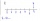Find four-digit numbers where all the digits are different. For numbers, the sum of the third and fourth digits is twice the sum of the first two digits, and the sum of the first and fourth digits is equal to the sum of the second and third digits. The di
• StoreOne meter of the textile was discounted by 2 USD. Now 9 m of textile cost as before 8 m. Calculate the old and new price of 1 m of the textile.
• We rollWe roll two dice A. - what is the probability that the sum of the falling numbers is at most 4 B. - is at least 10 C. - is divisible by 5?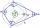What size have angles in quadrilateral (4-gon) if they are in a ratio of 8: 9: 10: 13?
• Digits A, B, CFor the various digits A, B, C is true: the square root of the BC is equal to the A and sum B+C is equal to A. Calculate A + 2B + 3C. (BC is a two-digit number, not a product).
• Three unknown numbers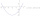The sum of of three numbers is 106. the first number is 10 more then the third. The second number is 2x the third. What are the numbers?
• Boys and girlsIf the ratio of male & female teachers is 2/5 & it is in proportion of the male & female students, how many are girls if there are 42 boys?
• Three numbers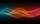The sum of the three numbers is 287. These numbers are in a ratio of 3: 7: 1/4. Find these numbers.
• A number 4A number is made up of two digits. The sum of the digits is 11. if the digits are interchanged, the original number is increase by 9. Find the original number
• Sum 10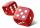What is the probability that two dice throw twice in a row will result the sum of 10?
• Digit sumThe digit sum of the two-digit number is nine. When we turn figures and multiply by the original two-digit number, we get the number 2430. What is the original two-digit number?